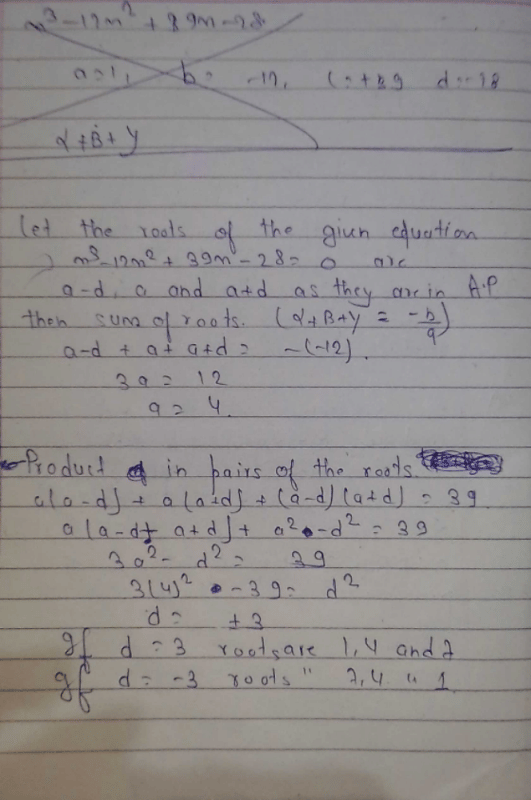find the zeroes of the polynomial f of x ...View courses related to this question Explore Class 10 courses
 Explore Class 10 coursesView courses related to this questionfind the zeroes of the polynomial f of x = x cube -12×Sq +39× - 28 ,if it is given that the zeroes are in AP.
?Abhi Prajapati1 Crore+ students have signed up on EduRev. Have you?

• ### if the sum of zeroes of the polynomial p(x)= kx²-5x²-11x-3 is 2 then k is e... more(Scan QR code)